Courses

# Test: Name Reaction- 3

## 15 Questions MCQ Test Organic Chemistry | Test: Name Reaction- 3

Description
This mock test of Test: Name Reaction- 3 for Chemistry helps you for every Chemistry entrance exam. This contains 15 Multiple Choice Questions for Chemistry Test: Name Reaction- 3 (mcq) to study with solutions a complete question bank. The solved questions answers in this Test: Name Reaction- 3 quiz give you a good mix of easy questions and tough questions. Chemistry students definitely take this Test: Name Reaction- 3 exercise for a better result in the exam. You can find other Test: Name Reaction- 3 extra questions, long questions & short questions for Chemistry on EduRev as well by searching above.
*Multiple options can be correct
QUESTION: 1

### The correct statement for osmium tetraoxide reagent is:

Solution:

A, B and C are correct. These are the properties of OSO4,

*Multiple options can be correct
QUESTION: 2

### In which of the following reaction the product will be aldehyde:

Solution:
*Multiple options can be correct
QUESTION: 3

### Which of the following reagent(s), cannot be used to carry out the following conversion: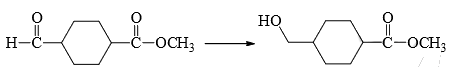Solution:

LiAlH4, Na/NH3 and B2H6/THF are simply too string reducing agents and will reduce even carboxylic acids. NaBH4 cannot reduce carboxylic acids and hence is the incorrect options.

*Multiple options can be correct
QUESTION: 4

Among the following, the reagent(s) which is/are suitable for the following conversion:​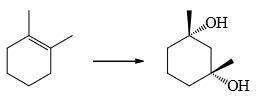Solution:
*Multiple options can be correct
QUESTION: 5

Which of the following combination(s) can be used to prepare alcohol given below: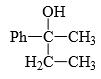Solution:

Option D produces 3-phenylpentan-3-ol while A, B, C produces our desired product.

*Multiple options can be correct
QUESTION: 6

Which of the following compound(s) is/are a suitable solvent for Grignard reaction:

Solution:
*Multiple options can be correct
QUESTION: 7

(Consider all step and intermediate) correct statement is/are: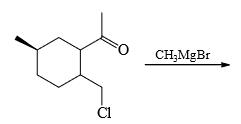Solution:

Since there is no hydrolysis happening, this is the final product.

Hence A, C and D are correct.

*Multiple options can be correct
QUESTION: 8

Choose the correct statement(s):

Solution:
*Multiple options can be correct
QUESTION: 9

Which of the following reagents give same product: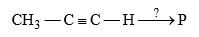Solution: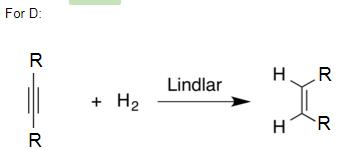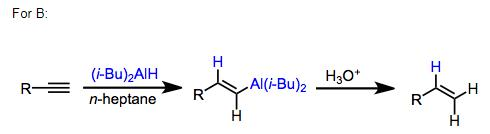Since they give the same product for these general reactions you can follow the same mechanism to get the required answer.

Hence B and D are the correct answers.

*Multiple options can be correct
QUESTION: 10

Which of the following reaction lead to increase in ring size in the product:

Solution:
*Multiple options can be correct
QUESTION: 11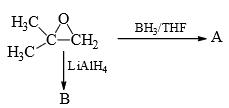Correct statement(s) is/are:

Solution:

Since LiAlH4 is stronger it will be able to reduce even the more hindered site and thus leaving the less hindered site for BH3/THF.

*Multiple options can be correct
QUESTION: 12

Which of the following reaction(s) is/are correct:

Solution:
*Multiple options can be correct
QUESTION: 13

Which of the following reaction(s) is/are correct:

Solution:
*Multiple options can be correct
QUESTION: 14

Which of the following is/are lead to ring formation:

Solution:
*Multiple options can be correct
QUESTION: 15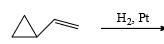Correct statement(s) is/are:

Solution: